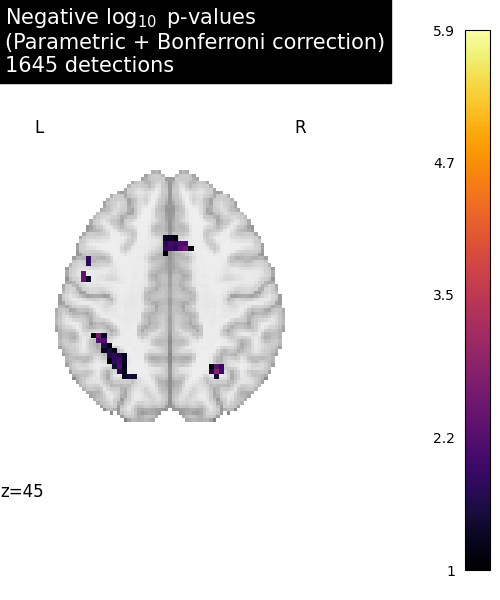# Massively univariate analysis of a calculation task from the Localizer dataset#

This example shows how to use the Localizer dataset in a basic analysis. A standard Anova is performed (massively univariate F-test) and the resulting Bonferroni-corrected p-values are plotted. We use a calculation task and 20 subjects out of the 94 available.

The Localizer dataset contains many contrasts and subject-related variates. The user can refer to the plot_localizer_mass_univariate_methods.py example to see how to use these.

Note

If you are using Nilearn with a version older than `0.9.0`, then you should either upgrade your version or import maskers from the `input_data` module instead of the `maskers` module.

That is, you should manually replace in the following example all occurrences of:

```from nilearn.maskers import NiftiMasker
```

with:

```from nilearn.input_data import NiftiMasker
```
```# Author: Virgile Fritsch, <virgile.fritsch@inria.fr>, May. 2014
import matplotlib.pyplot as plt
import numpy as np
from nilearn import datasets
from nilearn.image import get_data
```

```n_samples = 20
n_subjects=n_samples, legacy_format=False
)
tested_var = np.ones((n_samples, 1))
```

```nifti_masker = NiftiMasker(
smoothing_fwhm=5, memory="nilearn_cache", memory_level=1
)
cmap_filenames = localizer_dataset.cmaps
```

Anova (parametric F-scores)

```from sklearn.feature_selection import f_regression

# Center=False is used to not remove intercept
_, pvals_anova = f_regression(fmri_masked, tested_var, center=False)
pvals_anova[np.isnan(pvals_anova)] = 1
pvals_anova[pvals_anova > 1] = 1
neg_log_pvals_anova = -np.log10(pvals_anova)
neg_log_pvals_anova
)
```
```/home/yasmin/miniconda3/envs/nilearn-dev/lib/python3.10/site-packages/sklearn/utils/validation.py:1141: DataConversionWarning:

A column-vector y was passed when a 1d array was expected. Please change the shape of y to (n_samples, ), for example using ravel().
```

Visualization

```from nilearn.plotting import plot_stat_map, show

# Various plotting parameters
plotted_slice = 45
threshold = -np.log10(0.1)  # 10% corrected

# Plot Anova p-values
fig = plt.figure(figsize=(5, 6), facecolor="w")
display = plot_stat_map(
threshold=threshold,
display_mode="z",
cut_coords=[plotted_slice],
figure=fig,
)

)

title = (
"Negative \$\\log_{10}\$ p-values"
"\n(Parametric + Bonferroni correction)"